# The Root Test For Series Convergence

Vasudevan Harigovind, Yuanxin (Amy) Yang Alcocer, Christianlly Cena
• Author
Vasudevan Harigovind

Harigovind V R is a post graduate in mathematics from Amrita Vishwa Vidyapeetham. He has 1 year experience in teaching mathematics at college level.

• Instructor
Yuanxin (Amy) Yang Alcocer

Amy has a master's degree in secondary education and has been teaching math for over 9 years. Amy has worked with students at all levels from those with special needs to those that are gifted.

• Expert Contributor
Christianlly Cena

Christianlly has taught college Physics, Natural science, Earth science, and facilitated laboratory courses. He has a master's degree in Physics and is currently pursuing his doctorate degree.

Learn about the root test for the convergence of a series. Understand convergence and divergence of different series using the root test with examples. Updated: 02/22/2022

## Series Convergence Tests

A Series is a summation of infinitely many quantities given in a certain order. Consider the following expression:

$$\sum_{i=1}^{\infty} a_i$$

Any expression of this form is called a series. It's also represented as:

$$a_{1}+a_{2}+\cdots+a_{n}+\cdots$$

In connection to a series, the definition of a certain term is called sequence of partial sums.

Given a series of the form {eq}\sum_{i=1}^{\infty} a_i {/eq} a term in the sequence of partial sums {eq}S_{n} {/eq} is defined as the sum of first {eq}n {/eq} terms of the series. That is:

$$S_{n}\sum_{i=1}^{n} a_i$$

A series is said to converge to a certain real number, {eq}s {/eq}, if its sequence of partial sums converges to the real number {eq}s {/eq}. Such a series is called convergent.

When the sequence of partial sums diverges, the series is called divergent.

There are many tests to check whether a series converges or diverges. The most commonly used series convergence tests are:

• The Root Test
• The Ratio Test
• The Comparison Test
• The Integral Test

Knowing whether a series converges or diverges gives a definite way of working with it. When it's known that a series diverges, it is said that the series grows without bound and hence can be used in accordance. Different tests work in different manners on each series. To elaborate, some cases exist where one test fails to accomplish whether a certain series converges or diverges while using another gives us a definite answer.

## The Root Test

If you know that a series converges, then you can work further on it. But if it doesn't converge, then you can stop working on the series because you won't find an end to it. So how can you tell? Well, there's a test you can run.

The root test is a simple test that tests for absolute convergence of a series, meaning the series definitely converges to some value. This test doesn't tell you what the series converges to, just that your series converges.

The formal statement for the root test is:

For a series made up of terms an, define the limit as follows in this equation: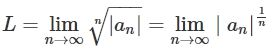We then keep the following in mind:

• If L < 1, then the series absolutely converges.
• If L > 1, then the series diverges.
• If L = 1, then the series is either divergent or convergent.

That last statement basically means that if you get 1 for your L then your answer is unknown. The root test can't tell whether your series converges or diverges.

Now, let's take a look at using the root test for a converging series, a diverging series, and an unknown or indeterminate series.An error occurred trying to load this video.

Try refreshing the page, or contact customer support.

Coming up next: Alternating Series: Definition & Examples

### You're on a roll. Keep up the good work!

Replay
Your next lesson will play in 10 seconds
• 0:04 The Root Test
• 1:19 Convergence Series
• 2:07 Divergent Series
• 2:30 One More Example
• 3:04 Lesson Summary
Save Save

Want to watch this again later?

Timeline
Autoplay
Autoplay
Speed Speed

## The Root Test for Series Convergence

The root test for series convergence states that:

Let {eq}\sum_{i=1}^{\infty} a_{i} {/eq} be a series of real numbers. Define {eq}L {/eq} as the limit:

$$L=\lim_{n\to \infty}\sqrt[n]{|a_n|}=\lim_{n\to \infty}(|a_n|)^{\frac{1}{n}}$$

Then:

• {eq}L<1 {/eq} implies the series converges.
• {eq}L>1 {/eq} implies the series diverges.
• {eq}L=1 {/eq} implies the test fails.

So if the value of {eq}L {/eq} is less than or greater than one, then there is a definite answer to the question of convergence, but when it's equal to one the test fails to inform about the convergence of the series and thus needs some other method of determination.

Applying the root test to the sequence part of a power series gives us the interval of convergence of a power series.

The root test series is considered to be one of the strongest among all series convergence tests.

The proof for the root test is as follows:

Let us consider each case:

• {eq}L<1 {/eq}:

{eq}L\geq 0 {/eq} always holds as it is the limit of the sequence of positive numbers.

Since {eq}L<1 {/eq} choose {eq}r > 0 {/eq} such that {eq}0<L+r<1 {/eq}( such an {eq}r {/eq} always exists, for example consider {eq}r=\frac{1-L}{2} {/eq}.

Since the sequence {eq}|a_n|^{\frac{1}{n}} {/eq} converges to {eq}L {/eq} we can find {eq}N \in \mathbb{N} {/eq} such that:$$\forall n \geq N \\ |a_{n}|^{\frac{1}{n}}<L+r$$

Therefore we see that:

$$\forall n \geq N \\ |a_{n}|<(L+r)^n$$.

Hence we see that

$$\forall n \geq N \\ a_{n}<(L+r)^n$$

This, in turn, implies that

$$\sum_{n=N}^{\infty}a_{n}<\sum_{n=N}^{\infty}(L+r)^n$$

The series on the right-hand side converges as it's the geometric series with its common ratio of less than one.

Consequently the series {eq}\sum_{n=N}^{\infty}a_{n} {/eq} converges by comparison test (given two series, if the greater one converges then so does the lesser).

Now going back to the series we see that:

$$\sum_{n=1}^{\infty}a_{n}= \sum_{n=1}^{N-1}a_{n}+\sum_{n=N}^{\infty}a_{n}$$

The first term in the sum on the right-hand side is the sum of finitely many real numbers and the second is a convergent series, Hence our series {eq}\sum_{n=1}^{\infty}a_{n} {/eq} converges.

• {eq}L > 1 {/eq}

Given

$$|a_{n}|^\frac{1}{n} \to L>1, n \to \infty$$

Corresponding to this we see that there exists some neighborhood of zero which contains only finitely many terms of the sequence {eq}a_{n} {/eq}. Therefore {eq}a_{n} {/eq} can never converge to zero. If the series converges, the limit of its corresponding sequence terms should be zero. Therefore our series does not converge when {eq}L > 1 {/eq}.

The third case is discussed in the examples in the final section.

## How to Tell if a Series Converges or Diverges Using the Root Test

We will see how to tell whether a series converges or diverges using the following example:

Consider the series:

$$\sum_{n=1}^{\infty}\left(\frac{n^2+3n}{4n^2+5n}\right)^n$$

Applying the root test:

\begin{aligned} L&=\lim_{n\to\infty}\sqrt[n]{\left|\frac{n^2+3n}{4n^2+5n}\right|^n}\\&=\lim_{n\to\infty}{\left|\frac{n^2+3n}{4n^2+5n}\right|^n}^{\frac{1}{n}}\\&=\lim_{n\to\infty}{\left|\frac{n^2+3n}{4n^2+5n}\right|}\\&=\frac{1}{4}<1 \end{aligned}

Hence here {eq}L<1 {/eq} therefore the series converges.

Keep in mind how the powers are canceled off while applying limits. This is a very important aspect of the test, it works best when {eq}n^{th} {/eq} powers are present in the series term.

## Converging Series

First, let's look at a converging series. Here's the problem:

Use the root test to determine whether this series converges or diverges.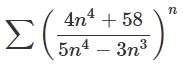To use the root test, you'll follow the statement for the root test and take the limit of the absolute value of the terms in the series taken to the 1 / n power like this series of equations appearing here: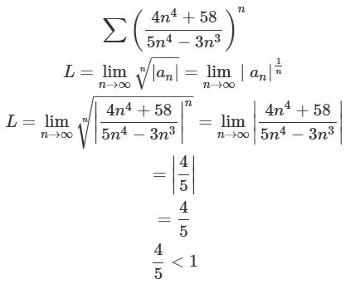The L is equal to 4 / 5, which is less than 1. Therefore, according to the root test, this series absolutely converges. Notice how the power of 1 / n was canceled since the series you are working with has an n power already. After that, you take the limit by plugging in infinity into your n variable and then canceling as much as you can, leaving you with 4 / 5.

To unlock this lesson you must be a Study.com Member.

Video Transcript

## The Root Test

If you know that a series converges, then you can work further on it. But if it doesn't converge, then you can stop working on the series because you won't find an end to it. So how can you tell? Well, there's a test you can run.

The root test is a simple test that tests for absolute convergence of a series, meaning the series definitely converges to some value. This test doesn't tell you what the series converges to, just that your series converges.

The formal statement for the root test is:

For a series made up of terms an, define the limit as follows in this equation:We then keep the following in mind:

• If L < 1, then the series absolutely converges.
• If L > 1, then the series diverges.
• If L = 1, then the series is either divergent or convergent.

That last statement basically means that if you get 1 for your L then your answer is unknown. The root test can't tell whether your series converges or diverges.

Now, let's take a look at using the root test for a converging series, a diverging series, and an unknown or indeterminate series.

## Converging Series

First, let's look at a converging series. Here's the problem:

Use the root test to determine whether this series converges or diverges.To use the root test, you'll follow the statement for the root test and take the limit of the absolute value of the terms in the series taken to the 1 / n power like this series of equations appearing here:The L is equal to 4 / 5, which is less than 1. Therefore, according to the root test, this series absolutely converges. Notice how the power of 1 / n was canceled since the series you are working with has an n power already. After that, you take the limit by plugging in infinity into your n variable and then canceling as much as you can, leaving you with 4 / 5.

To unlock this lesson you must be a Study.com Member.

• Activities
• FAQs

## Root Test for Convergence: True or False Activity

This activity will help assess your knowledge of the root test and how it is used as a test for convergence.

### Directions

Print or copy this page on blank paper. Then, write TRUE if the given statement is correct and FALSE if otherwise. Neatly write your answers on the appropriate blank space provided. Lastly, use the picture below as your reference.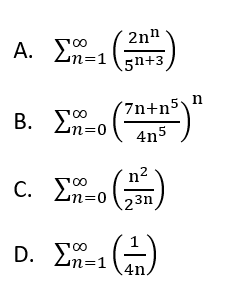__________ 1. In the root test, the convergence/divergence of the series is decided by the value of the limit L.

__________ 2. An L of positive infinity indicates that the series absolutely converges.

__________ 3. Taking the root test of series A will result in L equal to infinity.

__________ 4. Series A and D diverge.

__________ 5. The limit L can have positive and negative values.

__________ 6. A series diverges when its value gets closer and closer to a given limit or number.

__________ 7. Series D is either divergent or convergent.

__________ 8. Series B and C are both lower than one and would absolutely converge.

1. TRUE

2. FALSE, because the correct statement is: An L of positive infinity indicates that the series diverges.

3. TRUE

4. FALSE, because the correct statement is: Only series A diverges.

5. FALSE, because the correct statement is: The limit L can only have positive values.

6. FALSE, because the correct statement is: A series converges when its value gets closer and closer to a given limit or number.

7. TRUE

8. TRUE

### Solution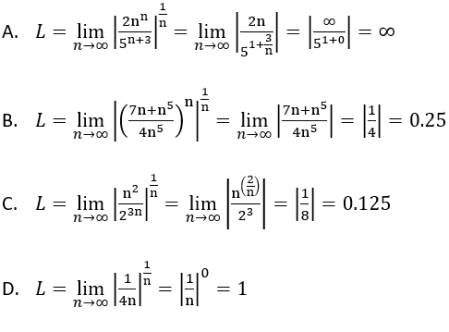#### Does the root test show absolute convergence?

Yes, the root test shows that the series is absolutely convergent and also converges conditionally. We use the modulus of the series terms, therefore, it gives conclusive proof that series converges absolutely.

#### Can you use the root test to find the interval of convergence?

Yes, in the case of a power series, applying the root test to the sequence in the power series gives the interval of convergence. Here the L value determines the radius of the interval of convergence.

#### What is the difference between the ratio test and the root test?

The ratio test uses the ratio between successive terms of the sequence to determine the convergence of the series. The root test employs the root of the nth term to do the same.

#### When can you use the root test?

The root test is most effective when used in series with nth power terms. It is of little help if the series contains factorials.

#### What is the root test for convergence?

The root test states that given a series with the limit of the sequence of nth roots of the nth term of the sequence that forms the series is less than one, then the series converges. If the limit is greater than one then the series diverges, and if the limit is equal to one then the test is inconclusive.

### Register to view this lesson

Are you a student or a teacher?

Back

### Resources created by teachers for teachers

Over 30,000 video lessons & teaching resources‐all in one place.Video lessonsQuizzes & WorksheetsClassroom IntegrationLesson Plans

I would definitely recommend Study.com to my colleagues. It’s like a teacher waved a magic wand and did the work for me. I feel like it’s a lifeline.

Jennifer B.
TeacherCreate an account to start this course today
Used by over 30 million students worldwide